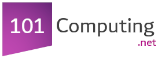Start

End
Create a program that asks the user to enter two whole numbers.
The program will then display which number is greater than the other.
num1 = int(input("Type a number:"))
num2 = int(input("Type another number:"))
if number1 > number2:
print(str(num1) + " is greater than " + str(num2))
else:
print(str(num2) + " is greater than " + str(num1))